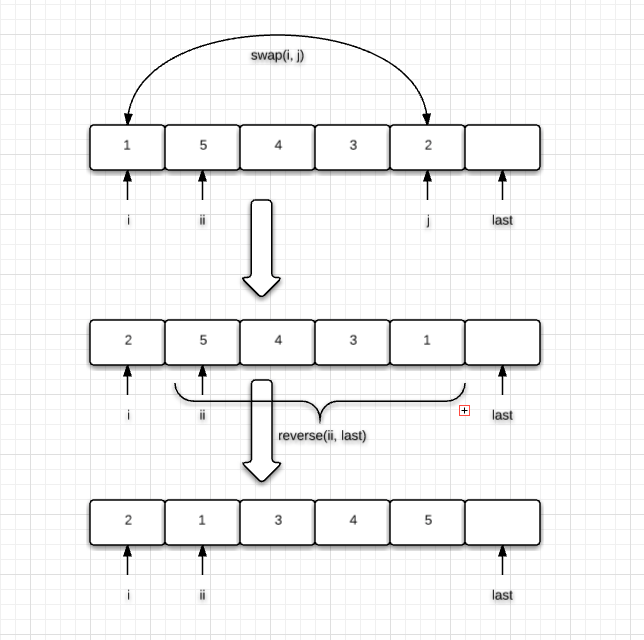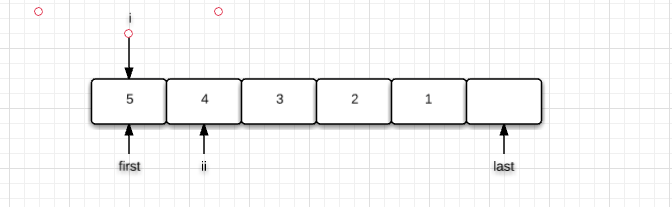# SGISTL源码探究-stl_algo.h中的排列算法

### 前言

``` stl_algo.h ```

``` next_permutation ```

``````#include <iostream>
#include <algorithm>
#include <string>
using namespace std;

int main()
{
string str;
cin >> str;
sort(str.begin(), str.end());
cout << str << endl;
while (next_permutation(str.begin(), str.end()))
{
cout << str << endl;
}
return 0;
}``````

``` next_permutation ```

``` [first, last) ```

``````/* 假如求解(A, B, C, D)的全排列
* 1. 将A固定,求(B, C, D)的全排列
* 2. 将B固定,求(A, C, D)的全排列
* 3. 将C固定,求(A, B, D)的全排列
* 4. 将D固定,求(A, B, C)的全排列
* 然后再依次将问题化小,分别求解
*/
template <class BidirectionalIterator>
void full_permutation(BidirectionalIterator first, BidirectionalIterator last)
{
if(first == last)
{
//输出该排列
}
else
{
BidirectionalIterator cur = first;
while(cur++ != last)
{
swap(*first, *cur);
++first;
full_permutation(first, last);
--first;
swap(*first, *cur);
}
}
}``````

### STL中的排列算法

``` SGISTL ```

``` next_permutation ```

``` prev_permutation ```
,即计算下一个排列以及前一个排列。

``` abcd ```
,它的下一个排列就是
``` abdc ```
,固定了
``` ab ```
,接着剩下的
``` cd ```

``` ac ```
,继续排列。

``` acbd ```

``` acdb ```

#### next_permutation

``` [first, last) ```

``````template <class BidirectionalIterator>
bool next_permutation(BidirectionalIterator first,
BidirectionalIterator last) {
//区间为空,返回false
if (first == last) return false;
BidirectionalIterator i = first;
++i;
//若区间只有1个元素,返回false
if (i == last) return false;
//i指向最后一个元素
i = last;
--i;

/* 从末尾往前扫,寻找第一对递增的元素(i和ii)
* 若找到了,从末尾往前找第一个比i大的元素,将两者互换
* 由于`[ii, last)`可能是递减序列,所以我们需要将它翻转成递增序列,从而导致所求的下一个排列的字典序大小尽量靠近本排列,然后返回true
* 如果第一步中直到扫到了first都还是没有递增的元素,那就证明已经没有下一个排列了,翻转整个序列,返回false
*/
for(;;) {
//i和ii指向相邻的两个元素
BidirectionalIterator ii = i;
--i;
//寻找相邻的递增元素
if (*i < *ii) {
//j指向last
BidirectionalIterator j = last;
//寻找第一个比i大的元素
while (!(*i < *--j));
//交换
iter_swap(i, j);
//翻转[ii, last)的序列
reverse(ii, last);
return true;
}
//当抵达first时还没有相邻的递增元素
if (i == first) {
reverse(first, last);
return false;
}
}
}``````

• 情况1:固定的元素不需要更改,对应图解如下• 情况2:需要更改固定的元素,对应图解如下• 情况3:没有下一个排列的序列了,对应图解如下#### prev_permutation

``` next_permutation ```

``````template <class BidirectionalIterator>
bool prev_permutation(BidirectionalIterator first,
BidirectionalIterator last) {
if (first == last) return false;
BidirectionalIterator i = first;
++i;
if (i == last) return false;
i = last;
--i;

for(;;) {
BidirectionalIterator ii = i;
--i;
//寻找相邻的递减元素
if (*ii < *i) {
BidirectionalIterator j = last;
//找到第一个比i小的元素
while (!(*--j < *i));
//交换i和j指向的元素
iter_swap(i, j);
//翻转
reverse(ii, last);
return true;
}
//直到扫到了first还未找到相邻的递减元素(即类似{1, 2, 3, 4, 5}这种情况),则退出
if (i == first) {
reverse(first, last);
return false;
}
}
}``````

### 小结

``` next_permutation ```

``` prev_permutation ```

``` stl_algo.h ```

``` sort ```

THE END